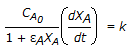# Chemical Engineering - Chemical Reaction Engineering

### Exercise :: Chemical Reaction Engineering - Section 1

36.

For an isothermal variable volume batch reactor, the following relation is applicable for a first order irreversible reaction.

 A. XA = k . t B.C. -ln(1 - XA) = kt D. εA . ln (1 - XA) = k . t

Explanation:

No answer description available for this question. Let us discuss.

37.

For a zero order chemical reaction, the

 A. half life period is directly proportion to the initial concentration of the reac-tants. B. plot of products concentration with time is a straight line through the origin. C. products concentration increases linerarly with time. D. all (a), (b) and (c).

Explanation:

No answer description available for this question. Let us discuss.

38.

BET apparatus

 A. measures the catalyst surface area directly. B. operates at very high pressure. C. is made entirely of stainless steel. D. none of these.

Explanation:

No answer description available for this question. Let us discuss.

39.

Radioactive decay follows __________ order kinetics.

 A. first B. second C. third D. zero.

Explanation:

No answer description available for this question. Let us discuss.

40.

The excess energy of reactants in a chemical reaction required to dissociate into products is termed as the __________ energy.

 A. activation B. potential C. binding D. threshold